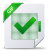# Algorithmes de type Expectation-Maximization en ligne pour l’estimation

Algorithmes de type Expectation-Maximization en ligne pour l’estimation

The Expectation Maximization (EM) algorithm is a versatile tool for model parameter estimation in latent data models. When processing large data sets or data stream however, EM becomes intractable since it requires the whole data set to be available at each iteration of the algorithm. In this contribution, a new generic online EM algorithm for model parameter inference in general Hidden Markov Model is proposed. This new algorithm updates the parameter estimate after a block of observations is processed (online). The convergence of this new algorithm is established, and the rate of convergence is studied showing the impact of the block-size sequence. An averaging procedure is also proposed to improve the rate of convergence. Finally, practical illustrations are presented to highlight the performance of these algorithms in comparison to other online maximum likelihood proce- dures. The Expectation Maximization (EM) algorithm is an iterative algorithm used to solve maximum likelihood estimation in HMM. The EM algorithm is generally simple to implement since it relies on complete data computa- tions. Each iteration is decomposed into two steps: the E-step computes the conditional expectation of the complete data log-likelihood given the observations and the M-step updates the parameter estimate based on this conditional expectation. In many situations of interest, the complete data likelihood belongs to the curved exponential family. In this case, the E-step boils down to the computation of the conditional expectation of the com- plete data suﬃcient statistic. Even in this case, except for simple models such as linear Gaussian models or HMM with ﬁnite state-spaces, the E-step is intractable and has to be approximated e.g. by Monte Carlo methods such as Markov Chain Monte Carlo methods or Sequential Monte Carlo methods (see [Carlin et al., 1992] or [Capp´e et al., 2005, Doucet et al., 2001] and the references therein).

However, when processing large data sets or data streams, the EM al- gorithm might become impractical. Online variants of the EM algorithm have been ﬁrst proposed for independent and identically distributed (i.i.d.) observations, see [Capp´ e et Moulines, 2009]. When the complete data like- lihood belongs to the cruved exponential family, the E-step is replaced by a stochastic approximation step while the M-step remains unchanged. The convergence of this online variant of the EM algorithm for i.i.d. observa- tions is addressed by [Capp´ e et Moulines, 2009]: the limit points are the stationary points of the Kullback-Leibler divergence between the marginal distribution of the observation and the model distribution. This new algorithm, called Block Online EM (BOEM) is derived in Sec- tion 6.2 together with an averaged version. Section 6.3 is devoted to practical applications: the BOEM algorithm is used to perform parameter inference in HMM where the forward recursions mentioned above are available explicitly. In the case of ﬁnite state-space HMM, the BOEM algorithm is compared to a gradient-type recursive maximum likelihood procedure and to the online EM algorithm of [Capp´e, 2011a]. The convergence of the BOEM algorithm is addressed in Section 6.4. The BOEM algorithm is seen as a perturbation of a deterministic limiting EM algorithm which is shown to converge to the stationary points of the limiting relative entropy (to which the true param- eter belongs if the model is well speciﬁed). The perturbation is shown to vanish (in some sense) as the number of observations increases thus implying that the BOEM algorithms inherits the asymptotic behavior of the limiting EM algorithm. Finally, in Section 6.5, we study the rate of convergence of the BOEM algorithm as a function of the block-size sequence. We prove that the averaged BOEM algorithm is rate-optimal when the block-size se- quence grows polynomially. All the proofs are postponed to Section 6.6; supplementary proofs and comments are provided in Appendix A.

Lire sur cLicours.com :  Software Engineering at VMware

The Block Online EM algorithm

Our algorithms update the parameter after processing a block of obser- vations. Nevertheless, the intermediate quantity S e, 2011a, Sec- tion 2.2] and [Del Moral et al., 2010b, Proposition 2.1] and can be applied either to ﬁnite state-space HMM or to linear Gaussian models. A Sequential Monte Carlo approximation to compute The classical theory of maximum likelihood estimation often relies on the assumption that the ”true” distribution of the observations belongs to the speciﬁed parametric family of distributions. In many cases, it is doubtful that this assumption is satisﬁed. It is therefore natural to investigate the convergence of the BOEM algorithms and to identify the possible limit for misspeciﬁed models i.e. when the observations Y are from an ergodic process which is not necessarily an HMM. In Section 6.3.1, the performance of the BOEM algorithm and its aver- aged version are illustrated in a truncated linear Gaussian model. In Sec- tion 6.3.2, the BOEM algorithm is compared to online maximum likelihood procedures in the case of ﬁnite state-space HMM.Télécharger le document complet